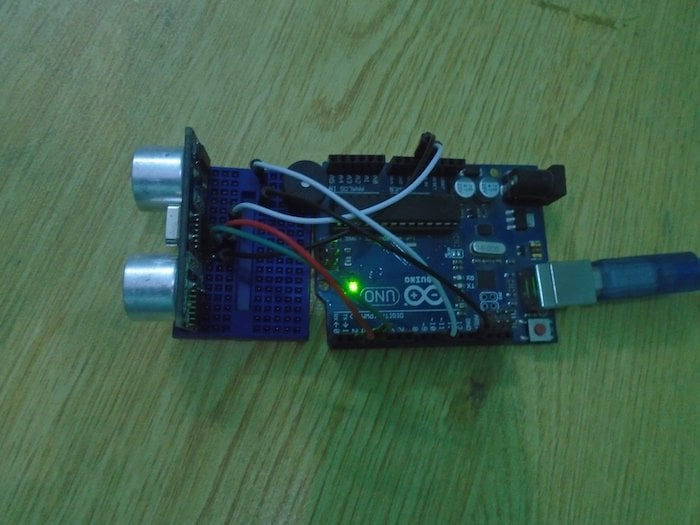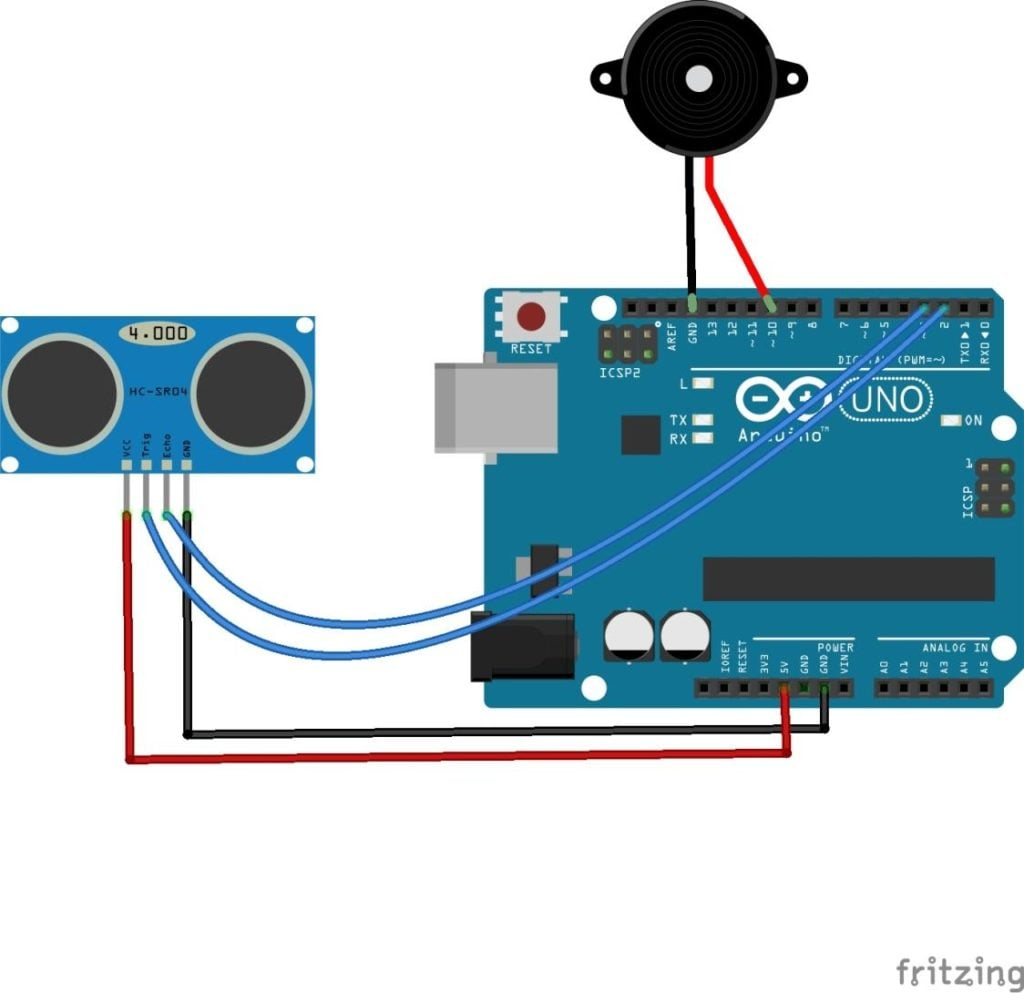Use an Arduino and an ultrasonic sensor to make a door alarm that sounds when someone comes within a certain distance.

In this project, we are going to make a door alarm system using the HC-SR04 ultrasonic sensor. The ultrasonic sensor used in this project is used as a distance sensor, it will tell us the distance at which the object is placed. Using this distance value, we will turn the buzzer on or off.### Circuit Diagram

The hardware part of this project is very easy to put together. First of all, make the connections for the ultrasonic sensor with the Arduino. The connections for the ultrasonic sensor with the Arduino are as follows:

• Connect VCC on the ultrasonic sensor to the 5V pin on the Arduino.
• Connect the Trig pin on the ultrasonic sensor to pin 2 on the Arduino.
• Connect the Echo pin on the ultrasonic sensor to pin 3 on the Arduino.
• Connect the GND on the ultrasonic sensor to GND on the Arduino.
After that, make the connections for the buzzer and the Arduino. Connect the positive pin on the buzzer with pin 10 on the Arduino and the buzzer's negative pin with the GND pin on the Arduino.### How Does the Arduino Door Alarm Work?

The ultrasonic sensor emits an ultrasonic wave from the trigger which comes back after hitting the object and it is received by the echo. The echo will then tell us the distance traveled in microseconds. To send an ultrasonic wave from the trigger, we will have to set the trigger high for 10us. This will send an 8 cycle sonic burst at 40 kHz which will hit the object and is then received by the echo.

We have got the time in microseconds but we need to calculate the distance. So, we will use the equation below.

S = v * t

We have the value of t and we know that the speed of a sound wave is 340m/s. We have to convert the speed of sound into cm/us to calculate the distance. The speed of sound in cm/us is 0.034cm/us. The equation now will become ...

S = (0.034 * t)

We will divide this equation by 2 because we only require the distance it takes to hit the object and not the distance it takes to hit the object and come back. So, the final equation will be

S = (0.035 * t)/2

We will get the distance value using the equation above and after that, we will set a value which will help us make the buzzer high or low.

### Code

```int trigger_pin = 2;

int echo_pin = 3;

int buzzer_pin = 10;

int time;

int distance;

void setup ( ) {

Serial.begin (9600);

pinMode (trigger_pin, OUTPUT);

pinMode (echo_pin, INPUT);

pinMode (buzzer_pin, OUTPUT);

}

void loop ( ) {

digitalWrite (trigger_pin, HIGH);

delayMicroseconds (10);

digitalWrite (trigger_pin, LOW);

time = pulseIn (echo_pin, HIGH);

distance = (time * 0.034) / 2;

if (distance <= 10)

{

Serial.println (" Door Open ");

Serial.print (" Distance= ");

Serial.println (distance);

digitalWrite (buzzer_pin, HIGH);

delay (500);

}

else {

Serial.println (" Door closed ");

Serial.print (" Distance= ");

Serial.println (distance);

digitalWrite (buzzer_pin, LOW);

delay (500);

}

}```

### Code Explanation

First of all, we initialized the trigger pin and echo for the ultrasonic sensor. We have connected the trigger pin to pin 2 on the Arduino and echo pin to pin 3 on the Arduino. Therefore, we initialized these pins in the code. Then we initialized the buzzer pin and after that, we initialized the variable which will help us in calculating the distance.

```int trigger_pin = 2;

int echo_pin = 3;

int buzzer_pin = 10;

int time;

int distance; ```

In the setup function, we declare the trigger pin as the output pin because we will send the ultrasonic wave through that pin. We made the echo pin as input pin because we will receive the ultrasonic wave through the echo.

```pinMode (trigger_pin, OUTPUT);

pinMode (echo_pin, INPUT);```

In the loop function, we set the trigger pin high for 10 us to send an ultrasonic wave and then we made the echo pin high to receive the ultrasonic wave. After that, we applied the equation to get the distance value.

```digitalWrite (trigger_pin, HIGH);

delayMicroseconds (10);

digitalWrite (trigger_pin, LOW);

time = pulseIn (echo_pin, HIGH);

distance = (time * 0.034) / 2;```

Then we made a condition that if the distance value is less than or equal to 10, then the buzzer will start to beep. If the distance value is greater than 10, then the buzzer will remain quiet.

```  if (distance <= 10)

{

Serial.println (" Door Open ");

Serial.print (" Distance= ");

Serial.println (distance);

digitalWrite (buzzer_pin, HIGH);

delay (500);

}```